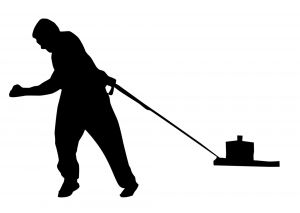Parents and Teachers: Support Ducksters by following us onor.

Science >> Physics for Kids

# Physics for Kids

## Laws of MotionA force is anything that can change the state of motion of an object, like a push or a pull. You use force when you push a letter on the computer keyboard or when you kick a ball. Forces are everywhere. Gravity acts as a constant force on your body, keeping you secure on planet Earth so you don't float away.To describe a force we use the direction and strength. For example when you kick a ball you are exerting force in a specific direction. That is the direction the ball will travel. Also, the harder you kick the ball the stronger the force you place on it and the farther it will go.

Laws of Motion

A scientist named Isaac Newton came up with three Laws of Motion to describe how things move scientifically. He also described how gravity works, which is an important force that affects everything.

First Law of Motion

The first law says that any object in motion will continue to move in the same direction and speed unless forces act on it.

That means if you kick a ball it will fly forever unless some sort of forces act on it! As strange as this may sound, it's true. When you kick a ball, forces start to act on it immediately. These include resistance or friction from the air and gravity. Gravity pulls the ball down to the ground and the air resistance slows it down.

Second Law of Motion

The second law states that the greater the mass of an object, the more force it will take to accelerate the object. There is even an equation that says Force = mass x acceleration or F=ma.

This also means that the harder you kick a ball the farther it will go. This seems kind of obvious to us, but having an equation to figure out the math and science is very helpful to scientists.

Third Law of Motion

The third law states that for every action, there is an equal and opposite reaction. This means that there are always two forces that are the same. In the example where you kicked the ball there is the force of your foot on the ball, but there is also the same amount of force that the ball puts on your foot. This force is in the exact opposite direction.Fun facts about Forces and Motion
• It is said that Isaac Newton got the idea for gravity when an apple fell off a tree and hit him on the head.
• Forces are measured in Newtons. This is after Isaac Newton, not fig newtons, even if they are tasty.
• Gases and liquids push out in equal forces in all directions. This is called Pascal's Law because it was discovered by the scientist Blaise Pascal.
• When you go upside down in a roller coaster loop-the-loop, a special kind of force called "centripetal force" keeps you in your seat and from falling out.
Activities

Forces and Motion Crossword Puzzle
Forces and Motion Word Search

More Physics Subjects on Motion, Work, and Energy

Science >> Physics for KidsFollow us onor